# RS Aggarwal Solutions for Class 9 Chapter 10: Quadrilaterals Exercise 10C

## RS Aggarwal Solutions for Class 9 Maths Exercise 10C PDF

RS Aggarwal solutions of Class 9 contains concepts which are tailored based on the abilities, grade levels and interests of students. The solutions are present in PDF format which can be downloaded for free by students. It has exercise wise answers which help students to achieve better marks in the board exams. All the questions are solved step by step along with the important formulas which are used. Conceptual knowledge is important in Mathematics as the topics are continued in Class 10 as well. RS Aggarwal Solutions for Class 9 Maths Chapter 10 Quadrilaterals Exercise 10C are provided here.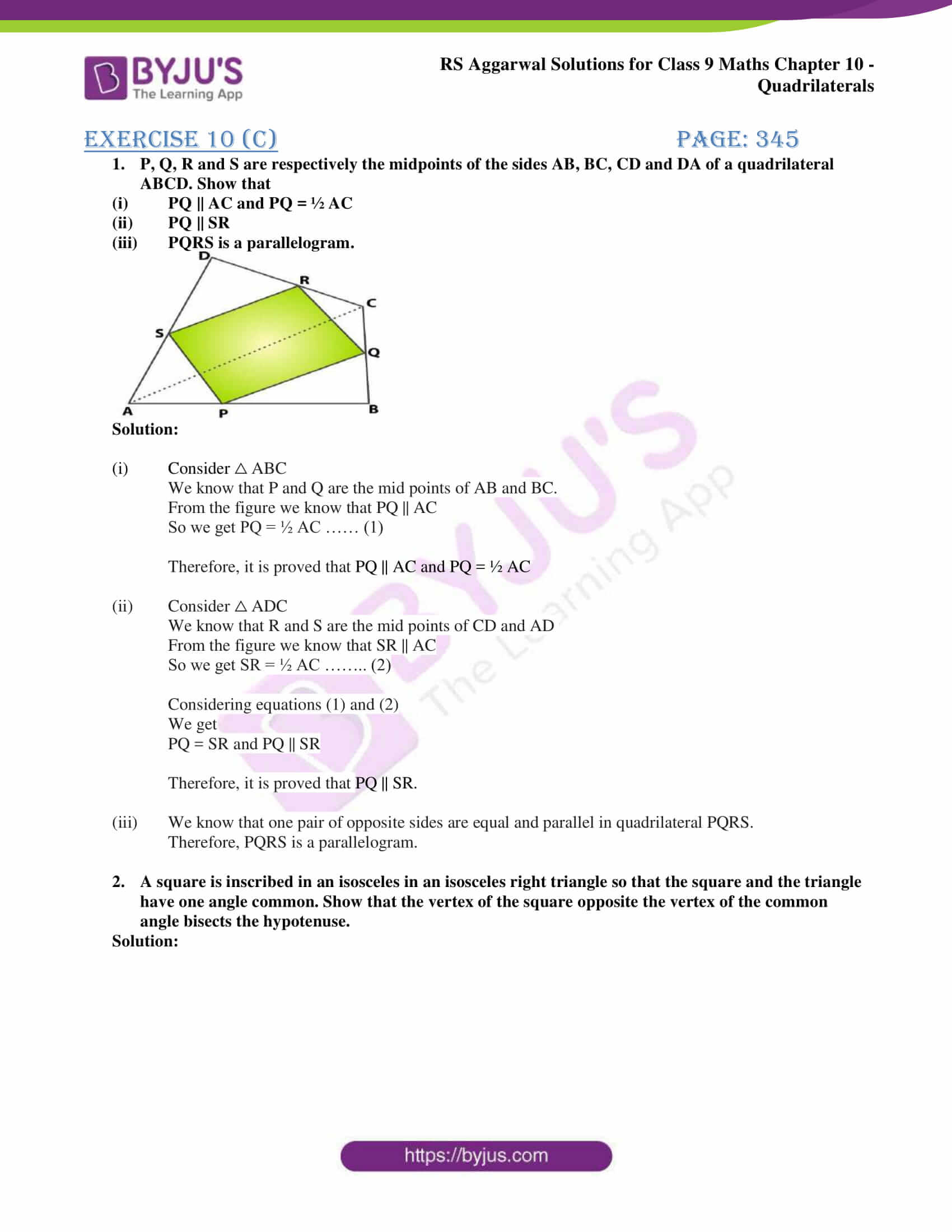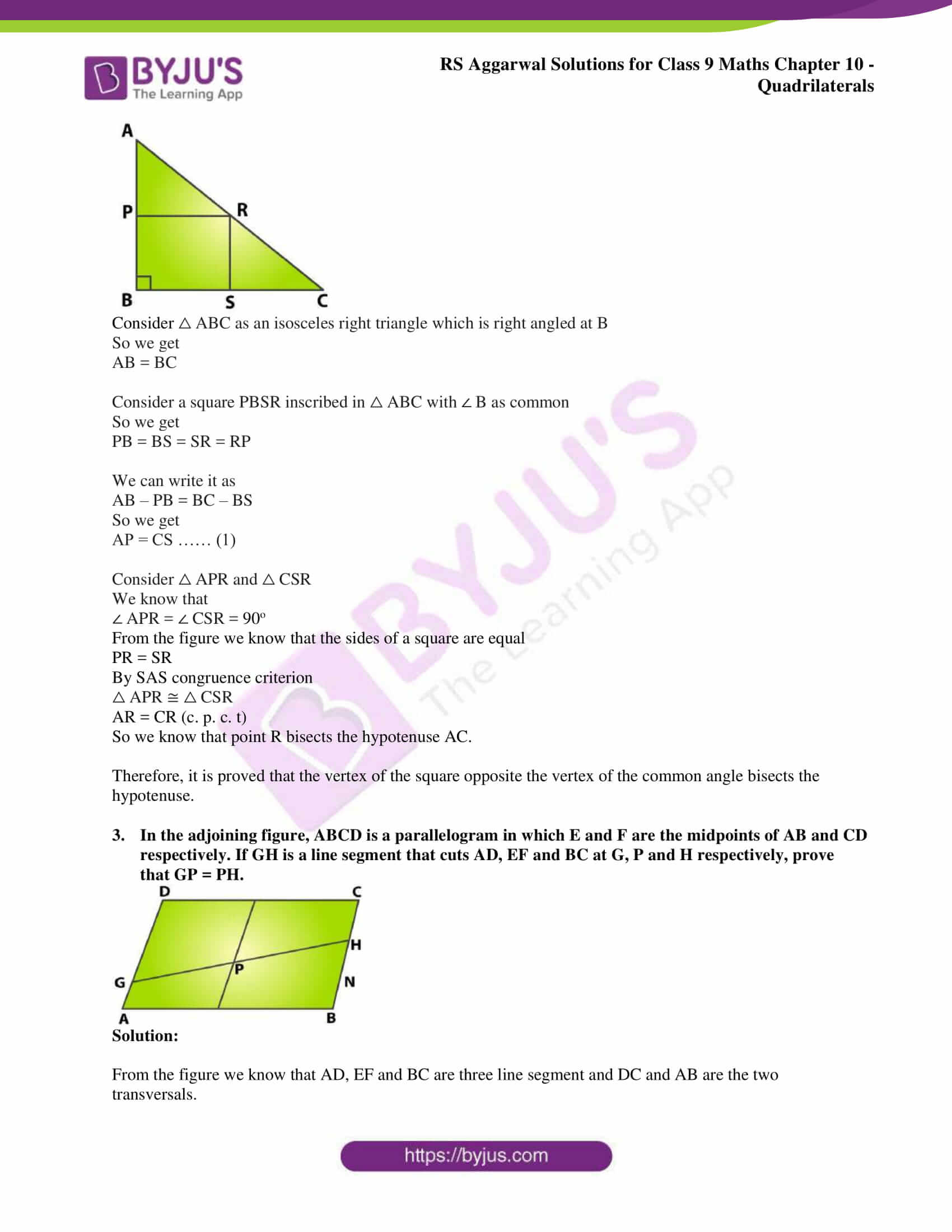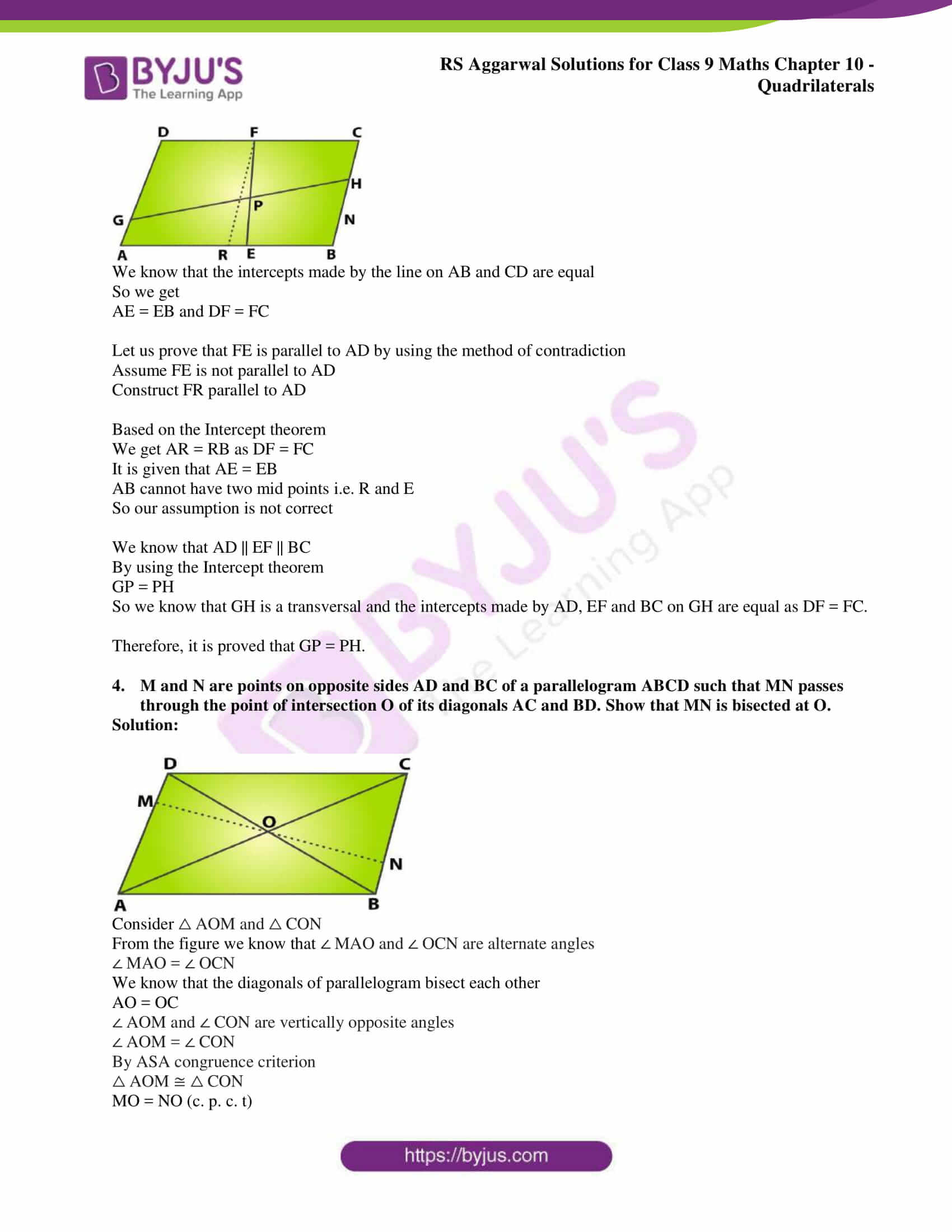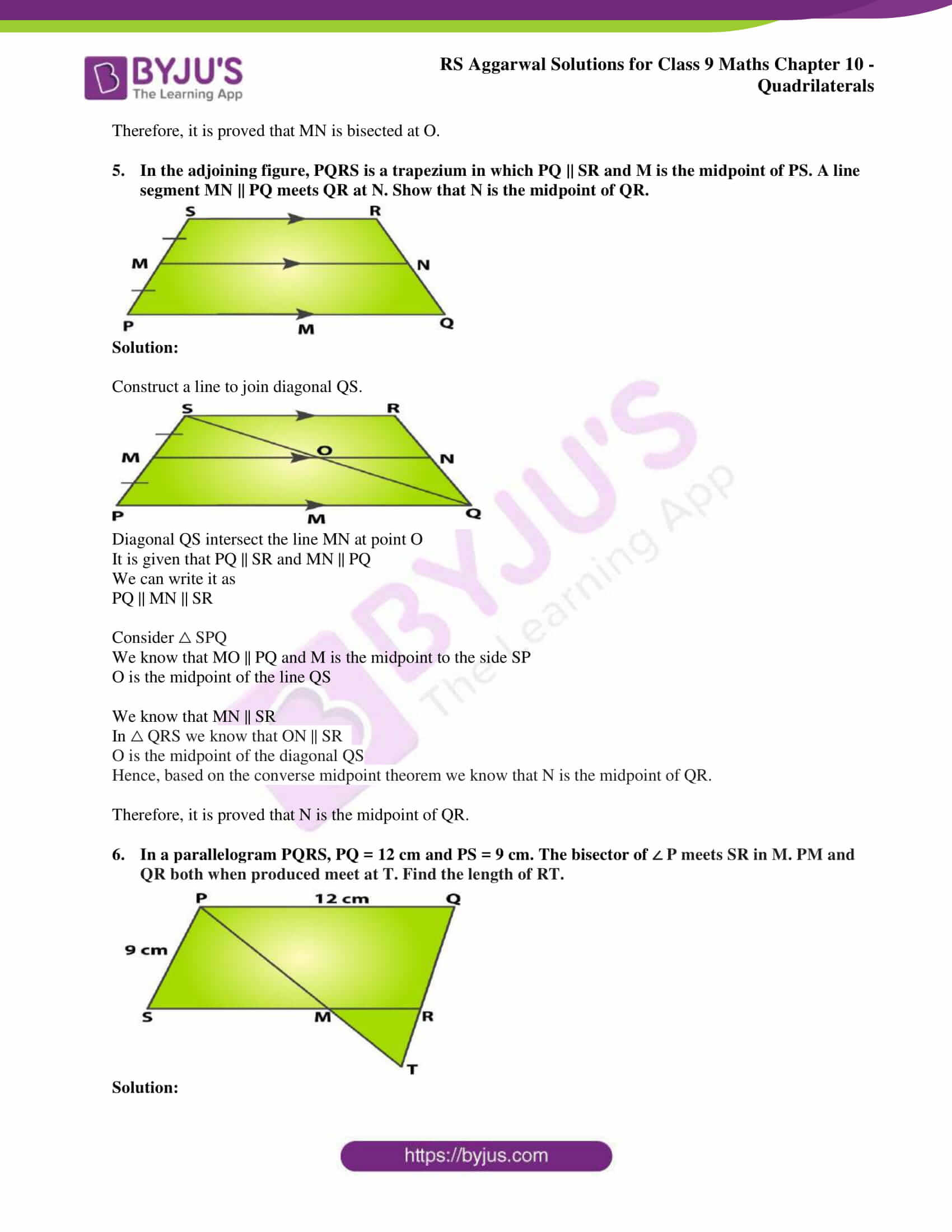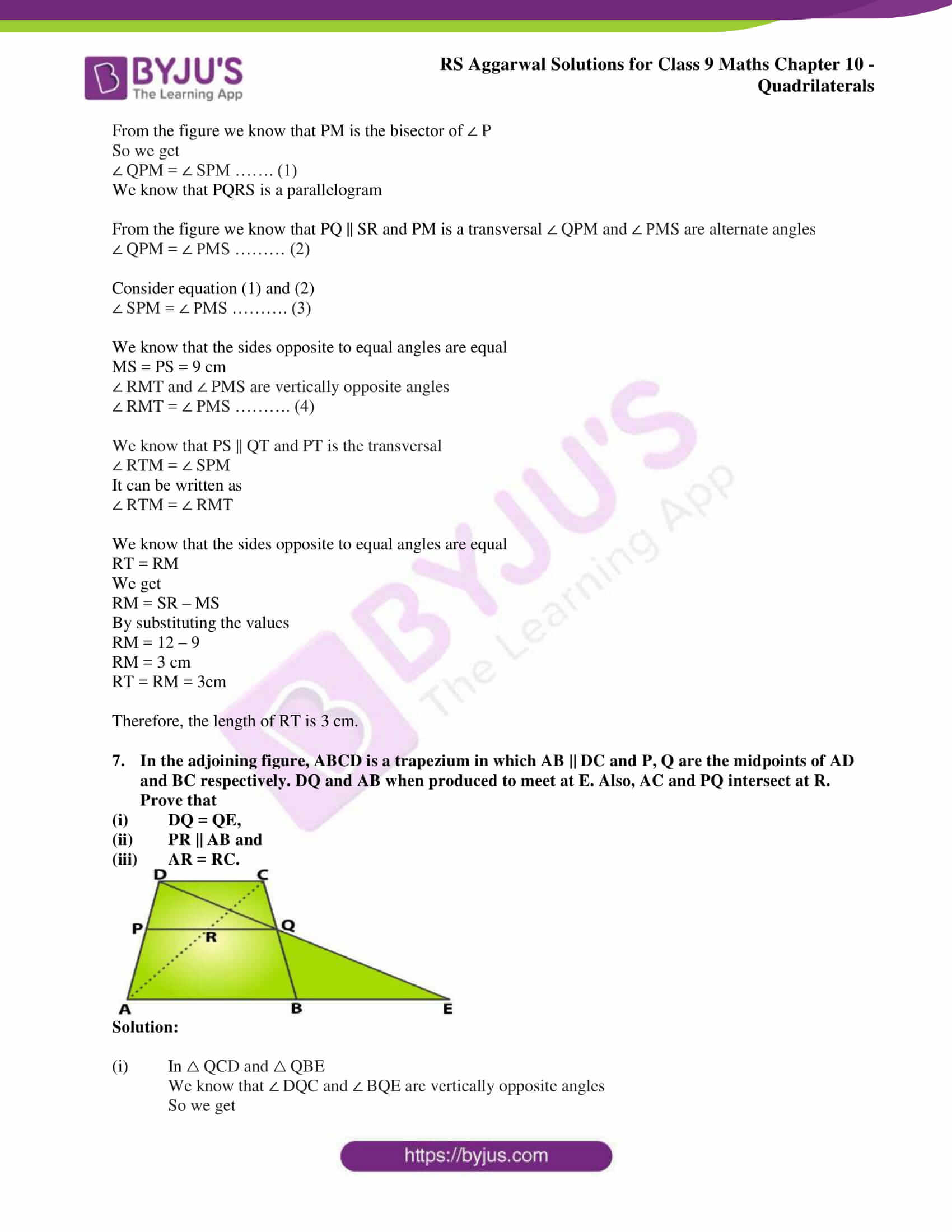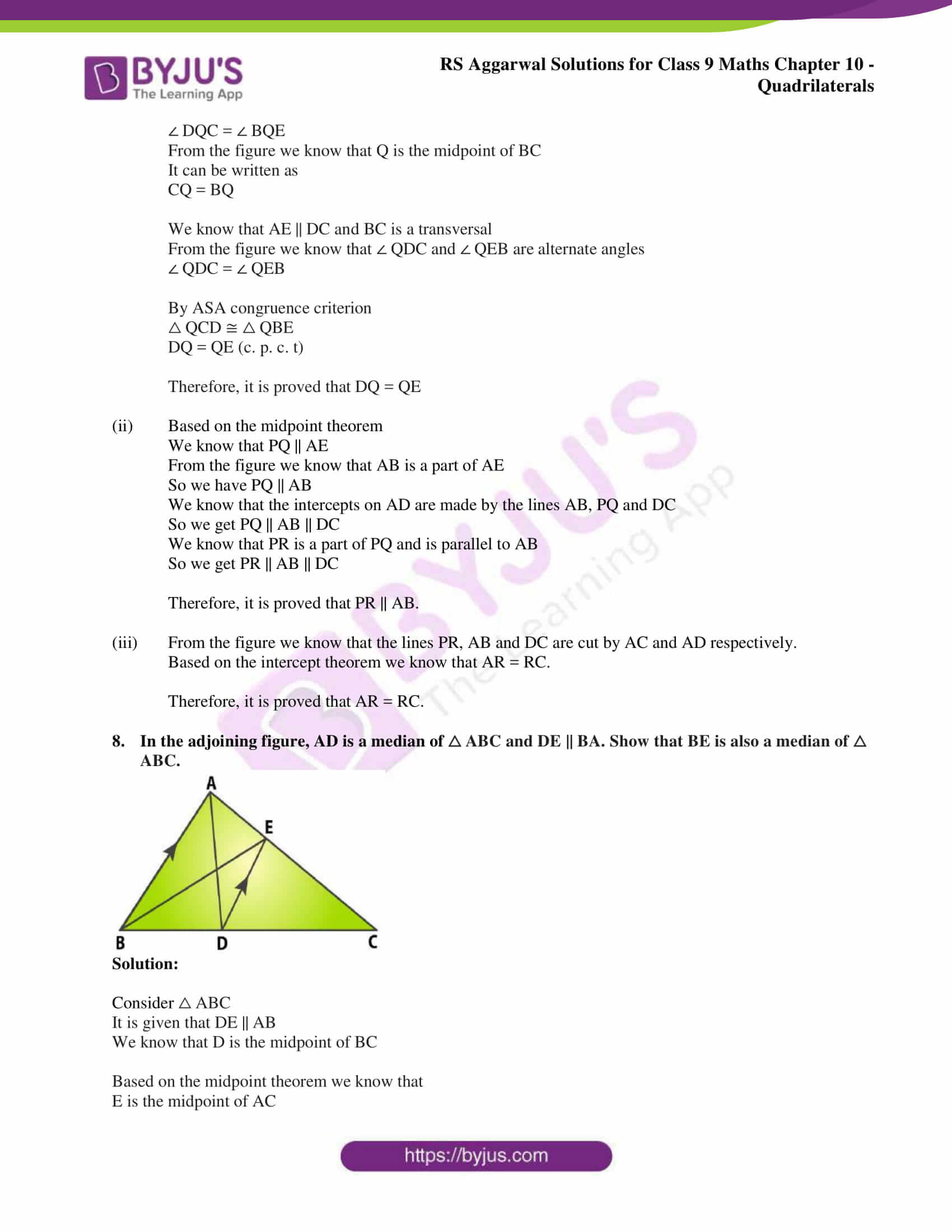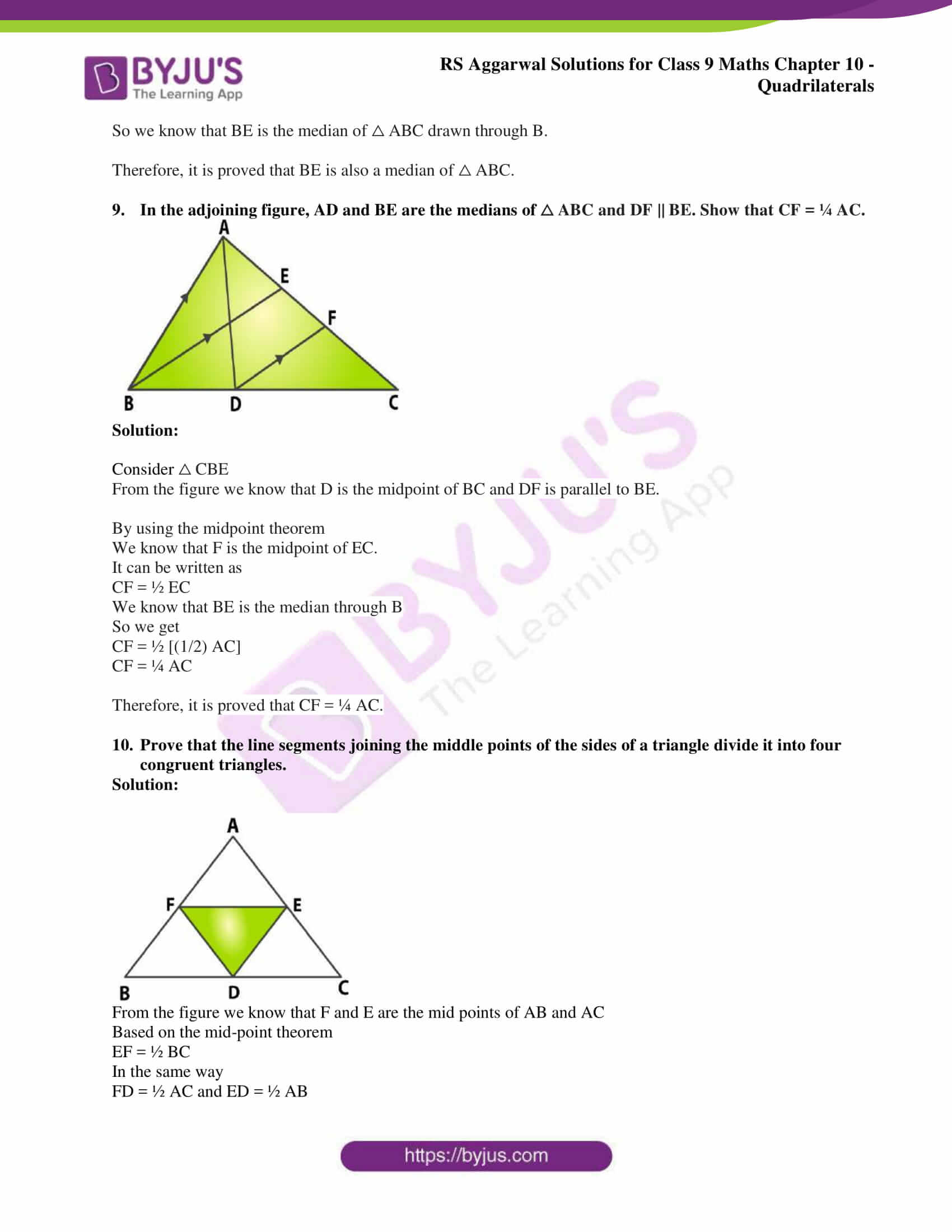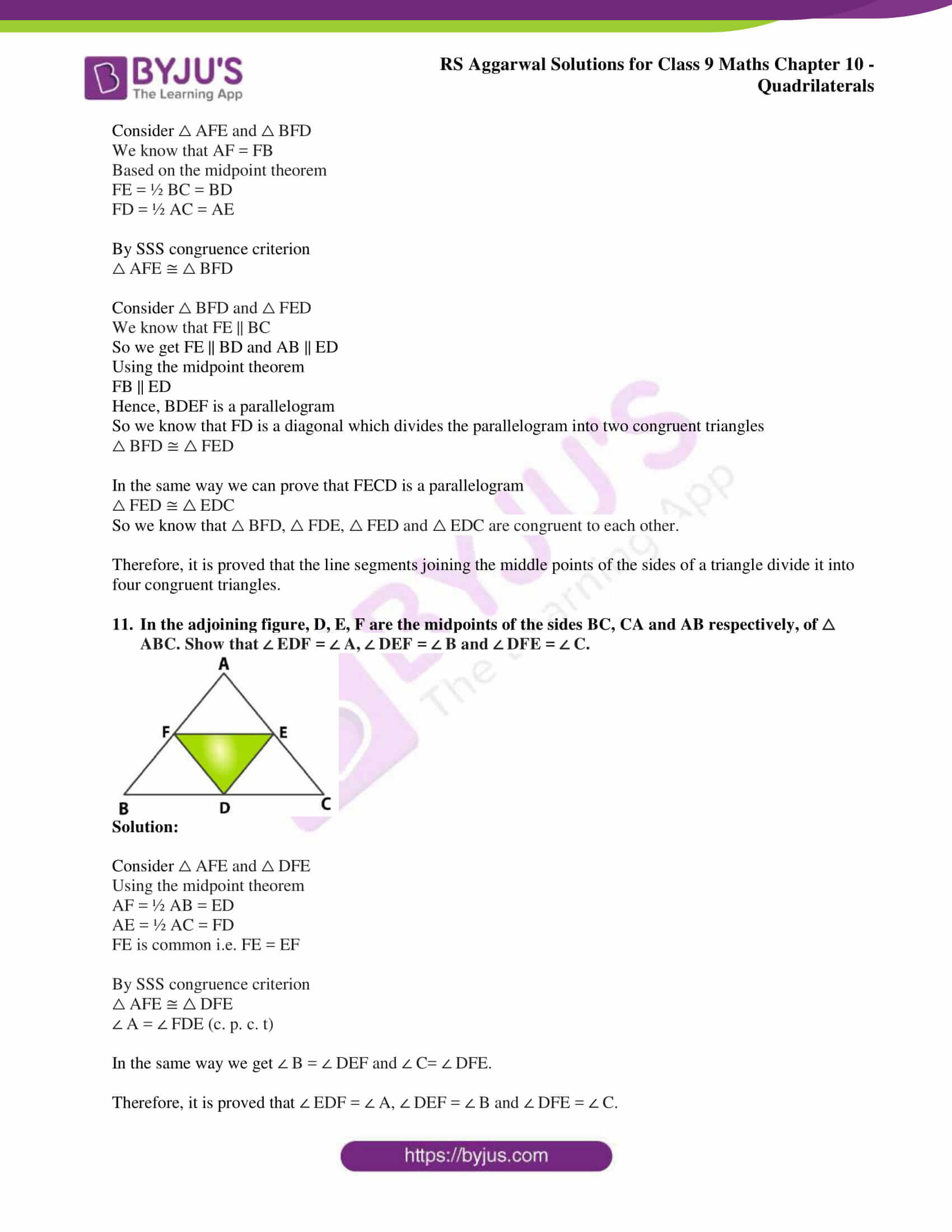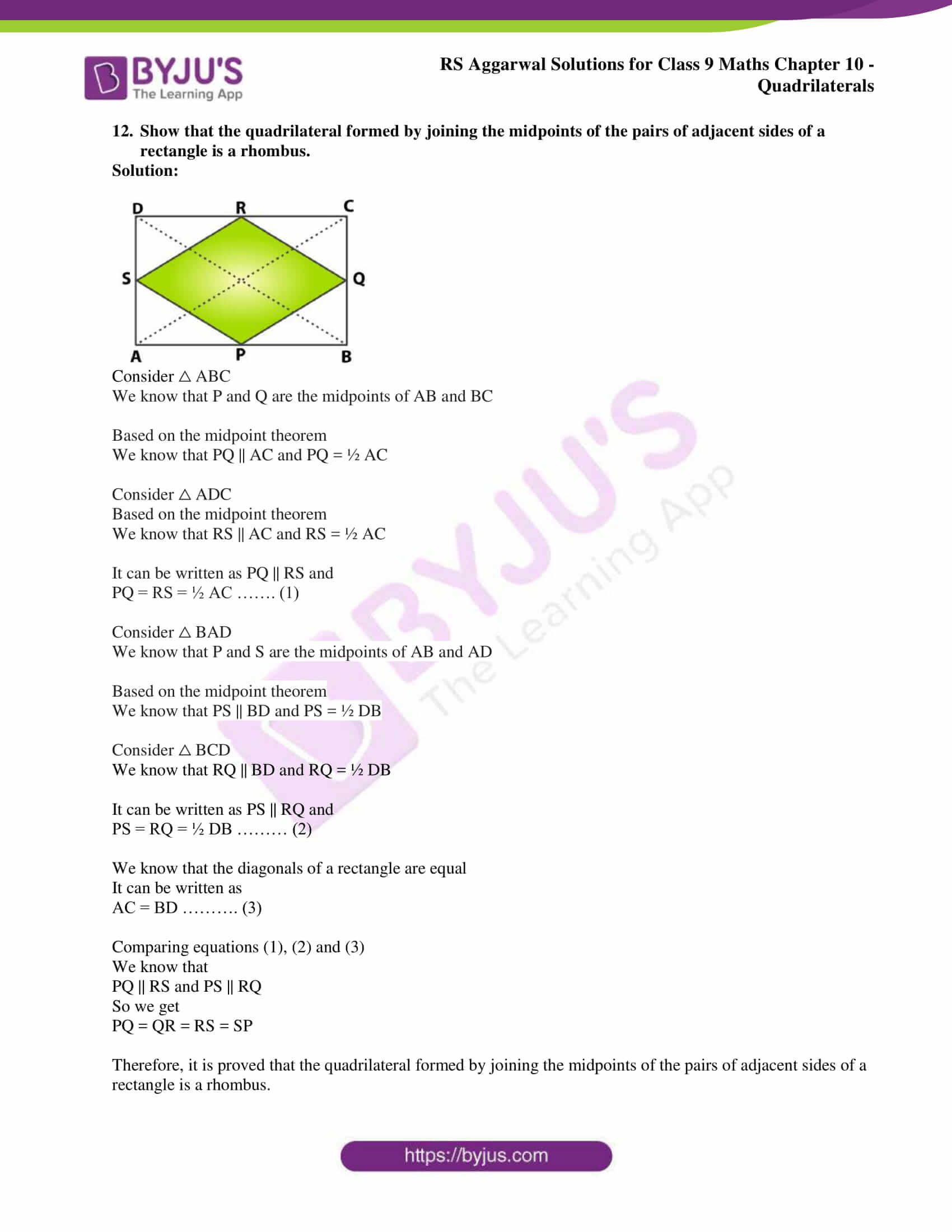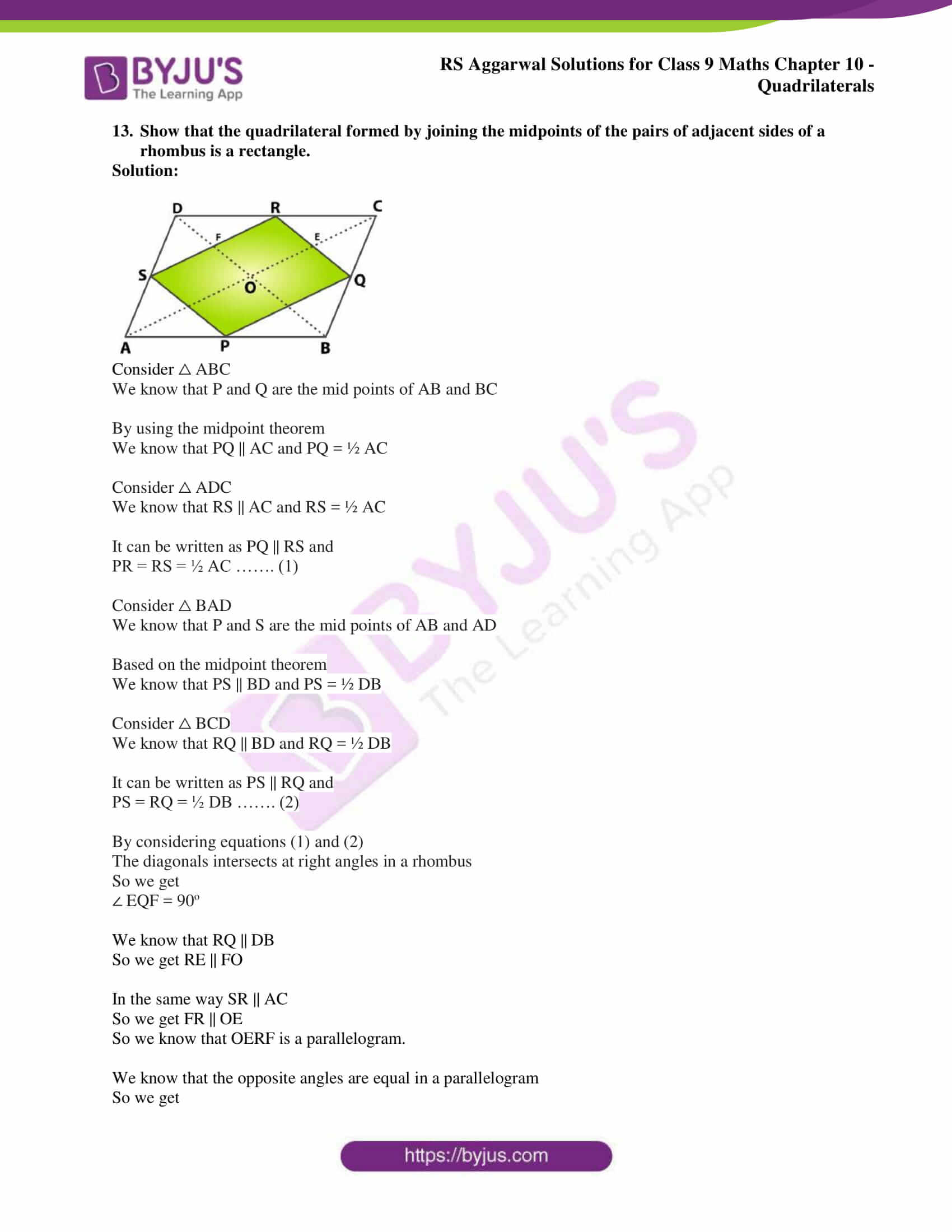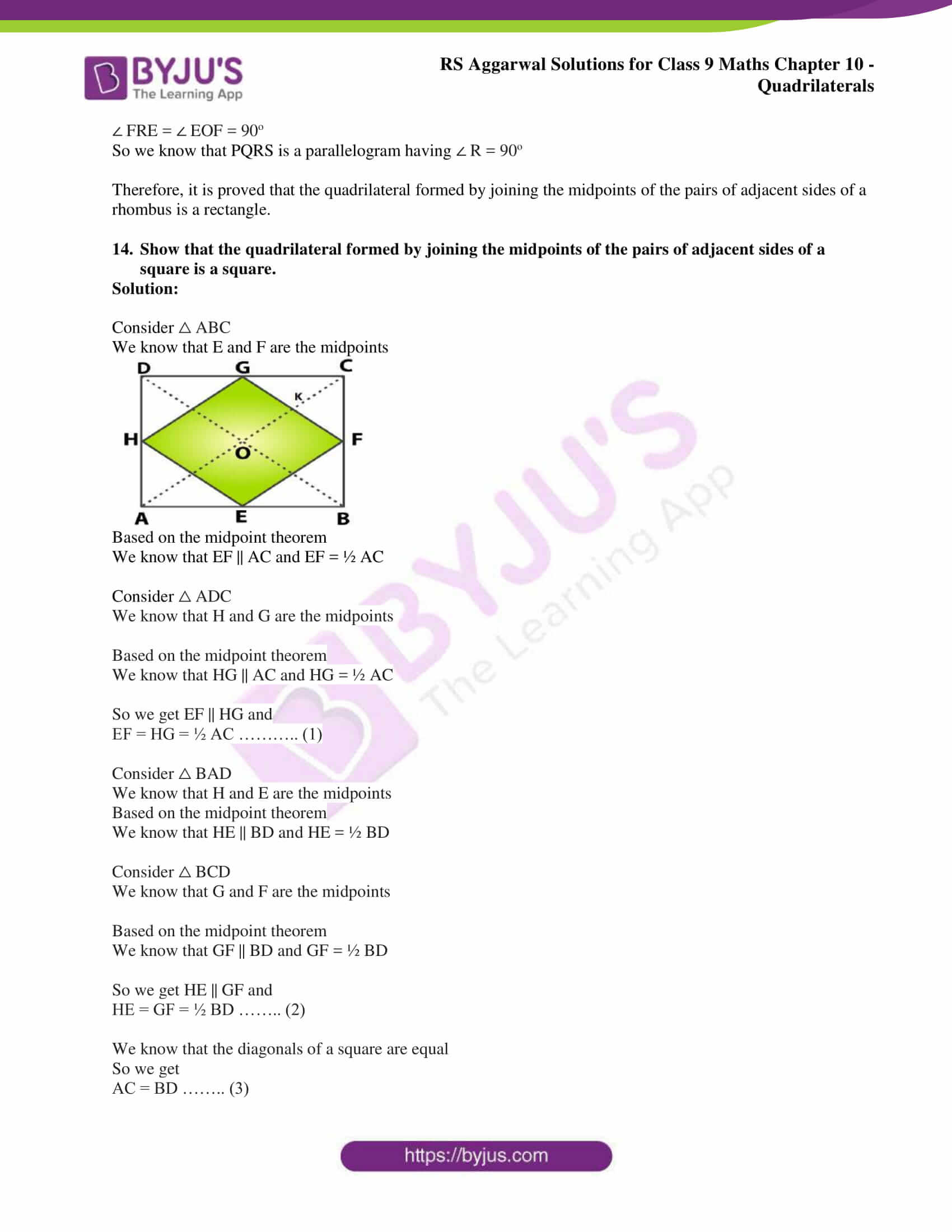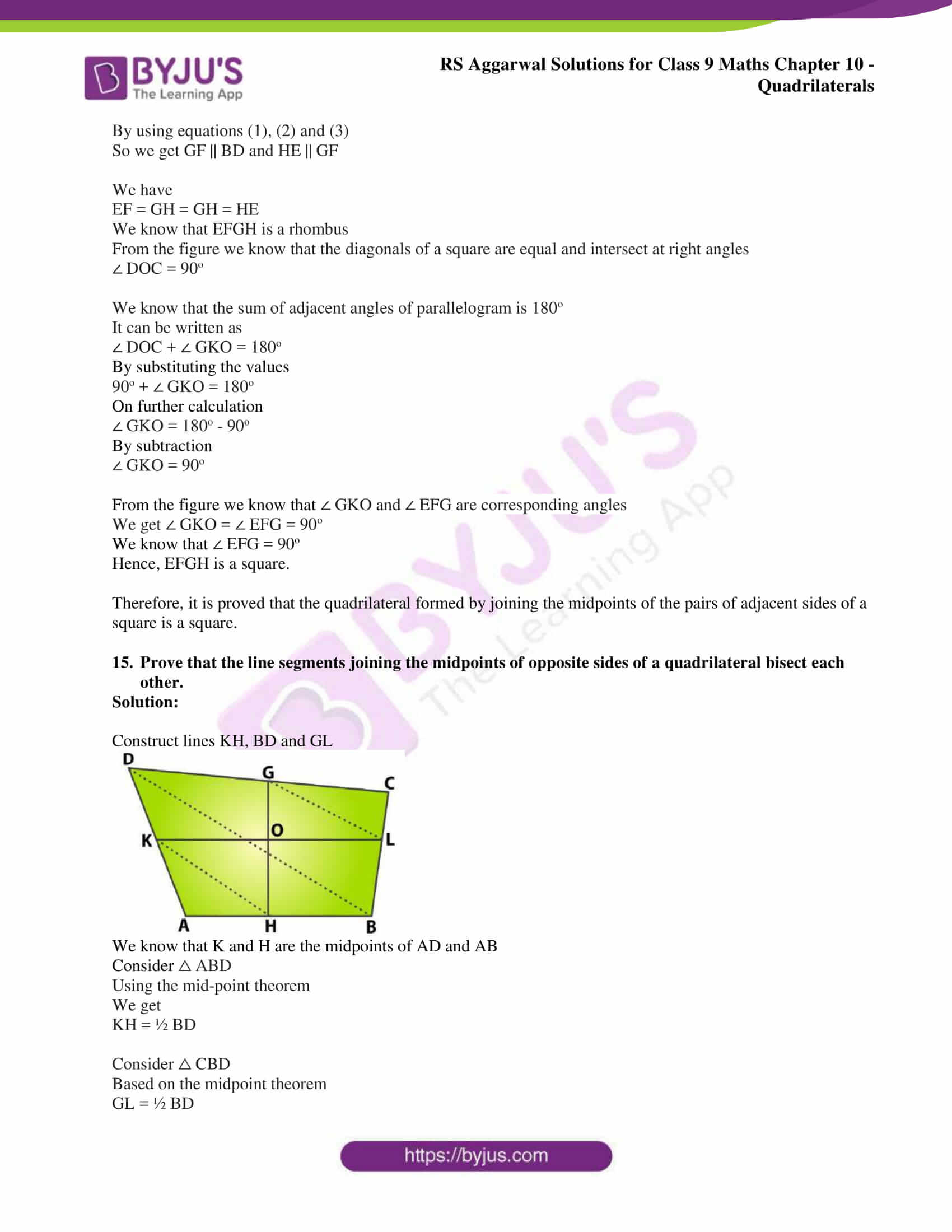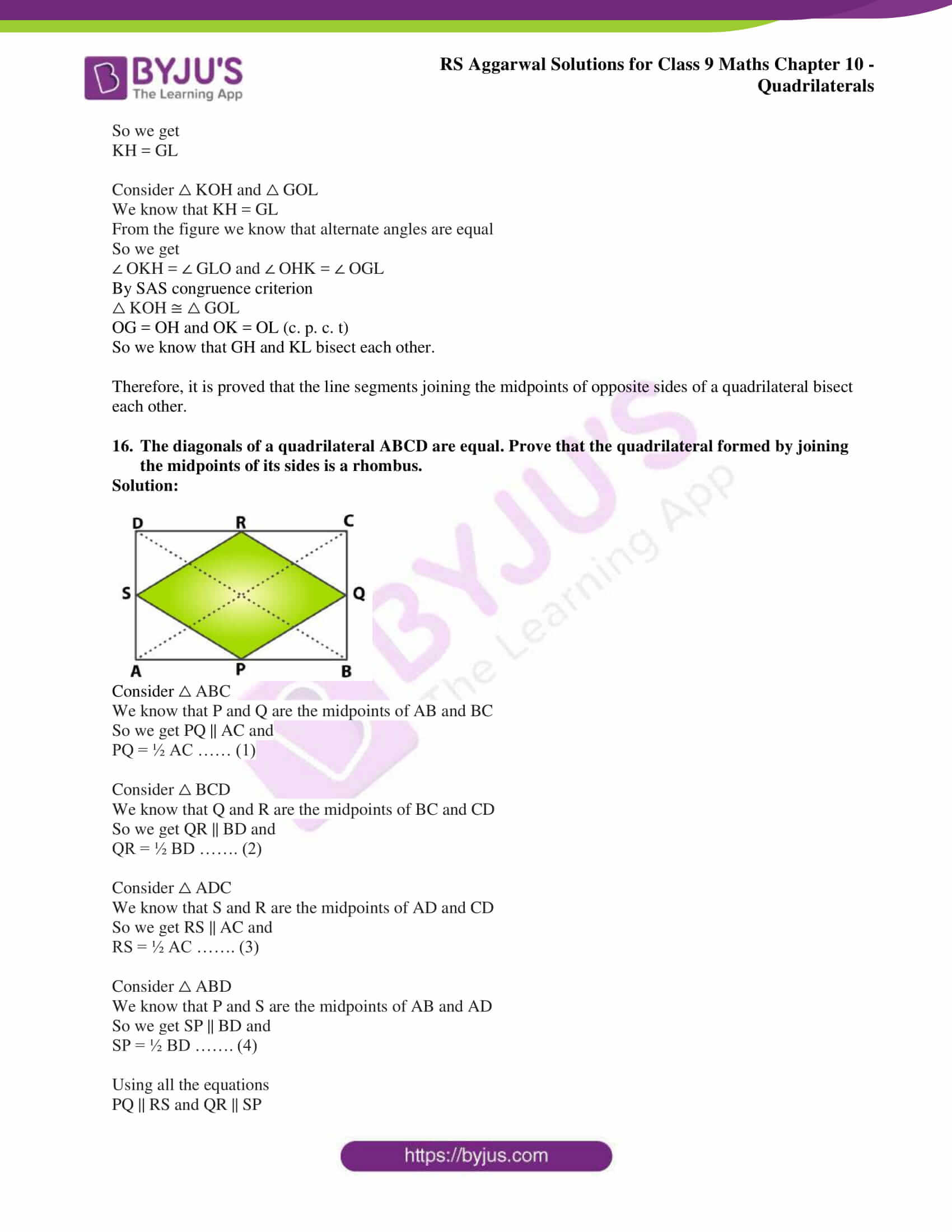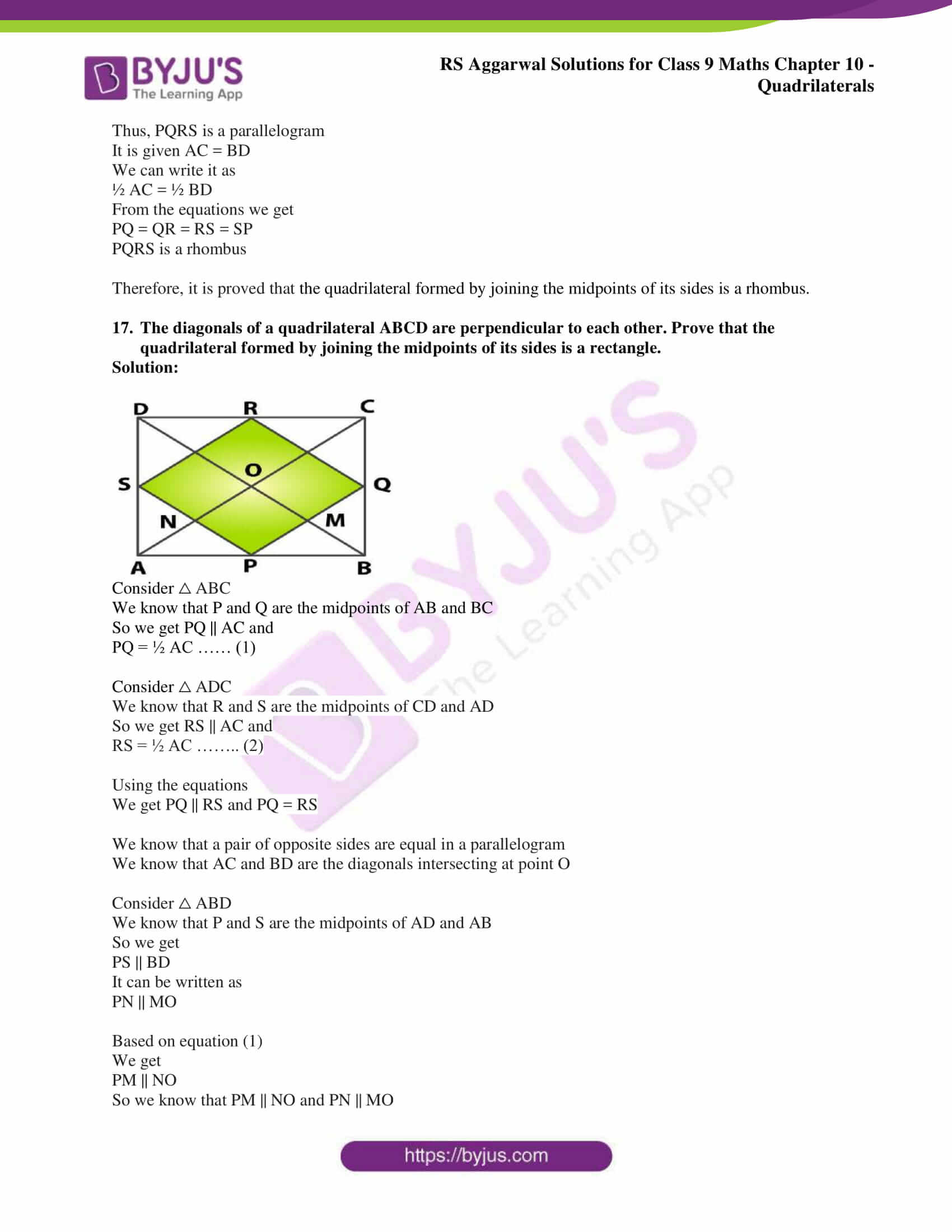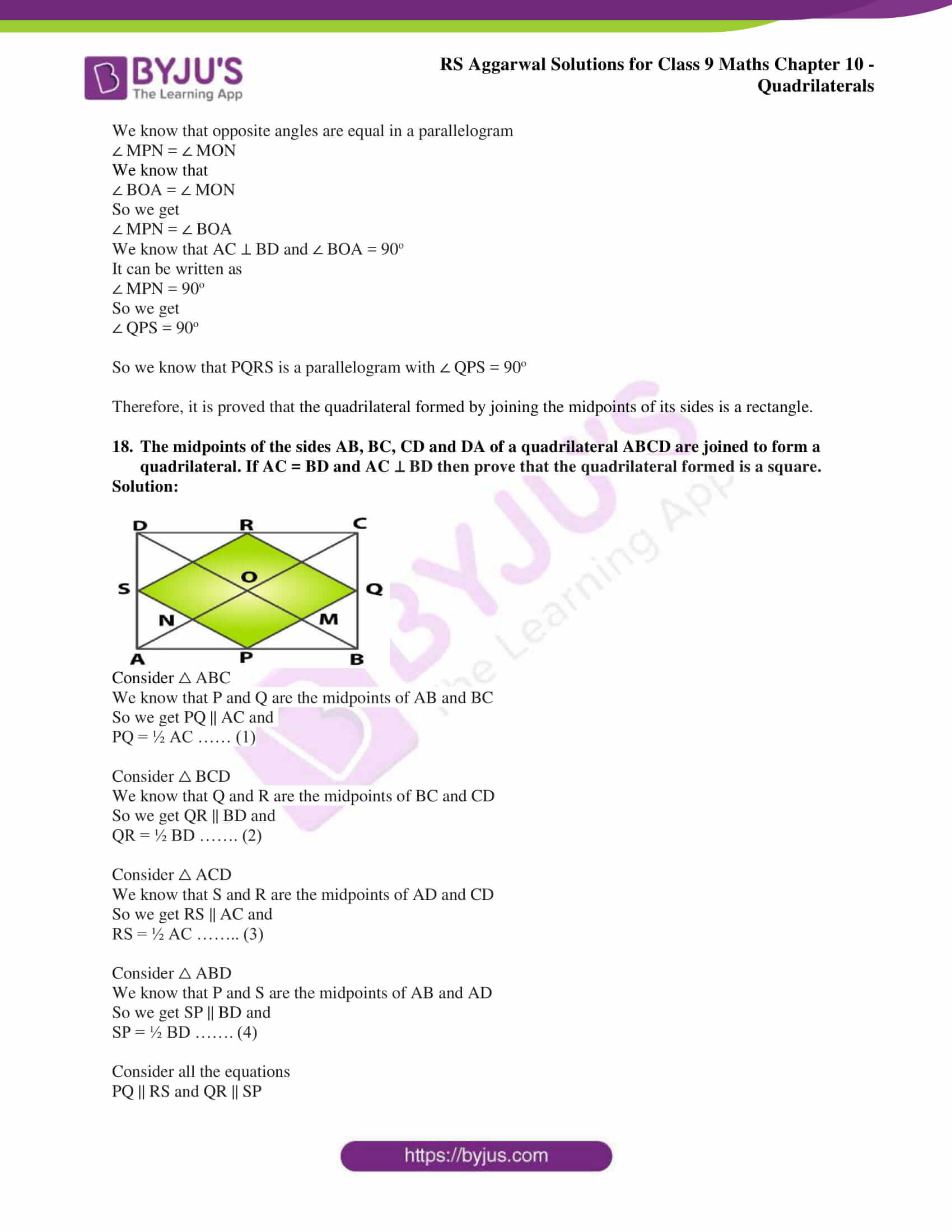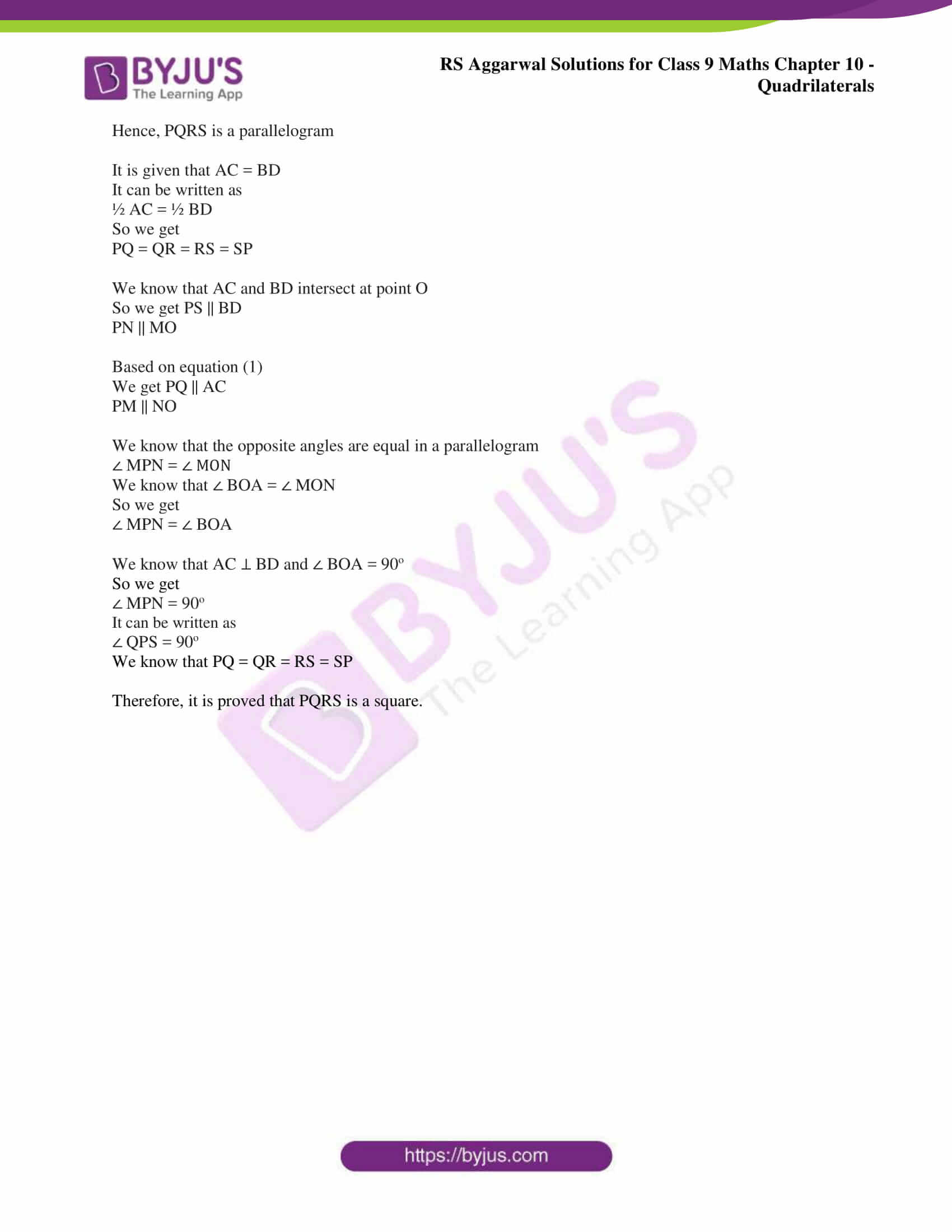## Access RS Aggarwal Solutions for Class 9 Chapter 10: Quadrilaterals Exercise 10C

1. P, Q, R and S are respectively the midpoints of the sides AB, BC, CD and DA of a quadrilateral ABCD. Show that

(i) PQ || AC and PQ = ½ AC

(ii) PQ || SR

(iii) PQRS is a parallelogram.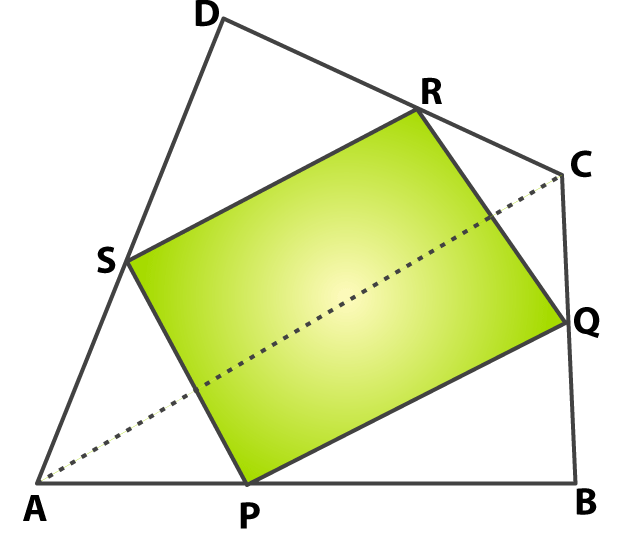Solution:

(i) Consider △ ABC

We know that P and Q are the mid points of AB and BC.

From the figure we know that PQ || AC

So we get PQ = ½ AC …… (1)

Therefore, it is proved that PQ || AC and PQ = ½ AC

We know that R and S are the mid points of CD and AD

From the figure we know that SR || AC

So we get SR = ½ AC …….. (2)

Considering equations (1) and (2)

We get

PQ = SR and PQ || SR

Therefore, it is proved that PQ || SR.

(iii) We know that one pair of opposite sides are equal and parallel in quadrilateral PQRS.

Therefore, PQRS is a parallelogram.

2. A square is inscribed in an isosceles in an isosceles right triangle so that the square and the triangle have one angle common. Show that the vertex of the square opposite the vertex of the common angle bisects the hypotenuse.

Solution: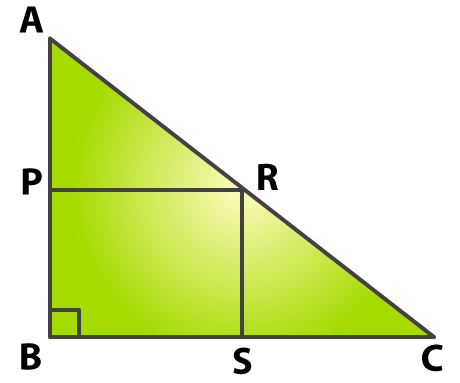Consider △ ABC as an isosceles right triangle which is right angled at B

So we get

AB = BC

Consider a square PBSR inscribed in △ ABC with ∠ B as common

So we get

PB = BS = SR = RP

We can write it as

AB – PB = BC – BS

So we get

AP = CS …… (1)

Consider △ APR and △ CSR

We know that

∠ APR = ∠ CSR = 90o

From the figure we know that the sides of a square are equal

PR = SR

By SAS congruence criterion

△ APR ≅ △ CSR

AR = CR (c. p. c. t)

So we know that point R bisects the hypotenuse AC.

Therefore, it is proved that the vertex of the square opposite the vertex of the common angle bisects the hypotenuse.

3. In the adjoining figure, ABCD is a parallelogram in which E and F are the midpoints of AB and CD respectively. If GH is a line segment that cuts AD, EF and BC at G, P and H respectively, prove that GP = PH.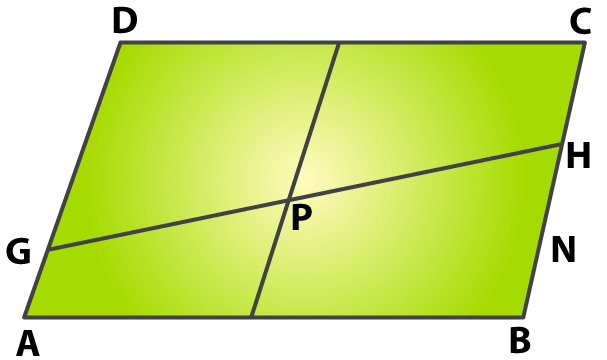Solution:

From the figure we know that AD, EF and BC are three line segment and DC and AB are the two transversals.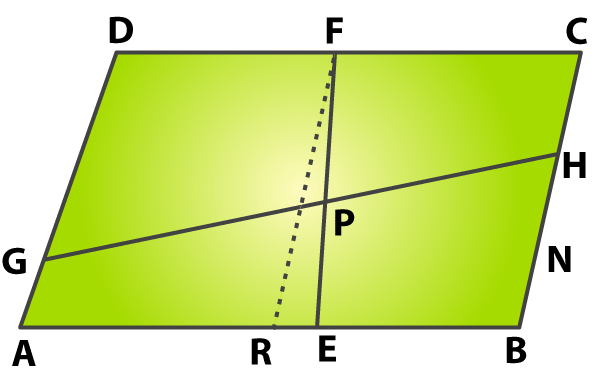We know that the intercepts made by the line on AB and CD are equal

So we get

AE = EB and DF = FC

Let us prove that FE is parallel to AD by using the method of contradiction

Assume FE is not parallel to AD

Based on the Intercept theorem

We get AR = RB as DF = FC

It is given that AE = EB

AB cannot have two mid points i.e. R and E

So our assumption is not correct

We know that AD || EF || BC

By using the Intercept theorem

GP = PH

So we know that GH is a transversal and the intercepts made by AD, EF and BC on GH are equal as DF = FC.

Therefore, it is proved that GP = PH.

4. M and N are points on opposite sides AD and BC of a parallelogram ABCD such that MN passes through the point of intersection O of its diagonals AC and BD. Show that MN is bisected at O.

Solution: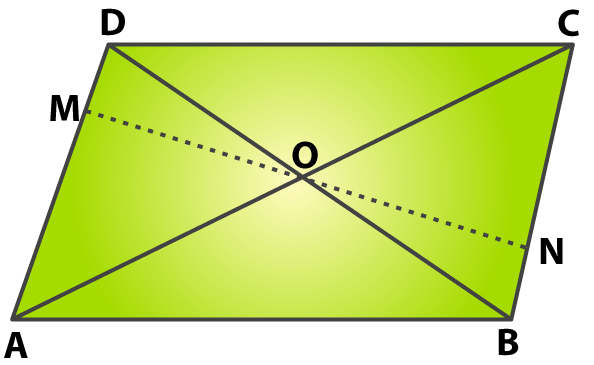Consider △ AOM and △ CON

From the figure we know that ∠ MAO and ∠ OCN are alternate angles

∠ MAO = ∠ OCN

We know that the diagonals of parallelogram bisect each other

AO = OC

∠ AOM and ∠ CON are vertically opposite angles

∠ AOM = ∠ CON

By ASA congruence criterion

△ AOM ≅ △ CON

MO = NO (c. p. c. t)

Therefore, it is proved that MN is bisected at O.

5. In the adjoining figure, PQRS is a trapezium in which PQ || SR and M is the midpoint of PS. A line segment MN || PQ meets QR at N. Show that N is the midpoint of QR.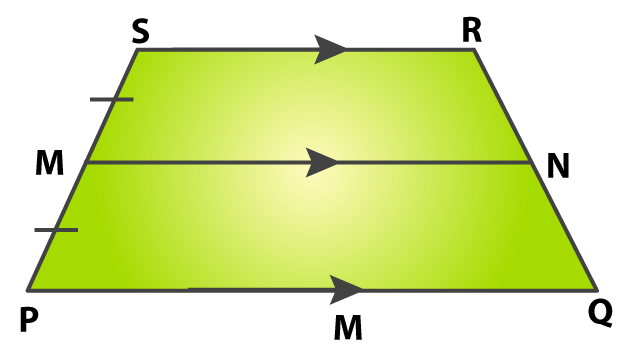Solution:

Construct a line to join diagonal QS.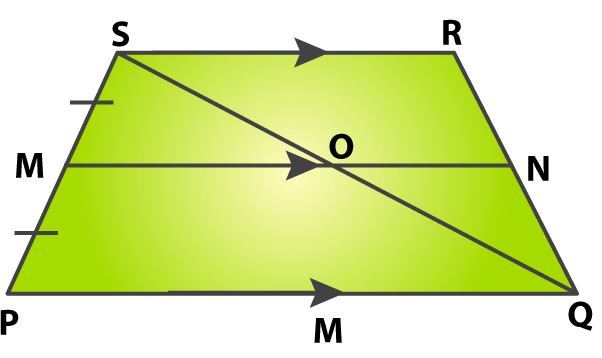Diagonal QS intersect the line MN at point O

It is given that PQ || SR and MN || PQ

We can write it as

PQ || MN || SR

Consider △ SPQ

We know that MO || PQ and M is the midpoint to the side SP

O is the midpoint of the line QS

We know that MN || SR

In △ QRS we know that ON || SR

O is the midpoint of the diagonal QS

Hence, based on the converse midpoint theorem we know that N is the midpoint of QR.

Therefore, it is proved that N is the midpoint of QR.

6. In a parallelogram PQRS, PQ = 12 cm and PS = 9 cm. The bisector of ∠ P meets SR in M. PM and QR both when produced meet at T. Find the length of RT.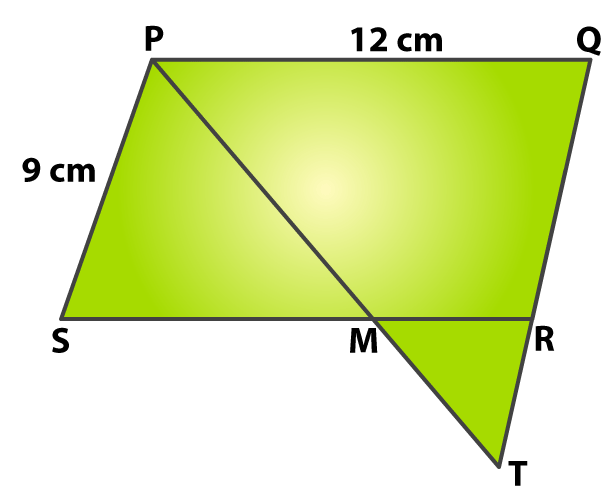Solution:

From the figure we know that PM is the bisector of ∠ P

So we get

∠ QPM = ∠ SPM ……. (1)

We know that PQRS is a parallelogram

From the figure we know that PQ || SR and PM is a transversal ∠ QPM and ∠ PMS are alternate angles

∠ QPM = ∠ PMS ……… (2)

Consider equation (1) and (2)

∠ SPM = ∠ PMS ………. (3)

We know that the sides opposite to equal angles are equal

MS = PS = 9 cm

∠ RMT and ∠ PMS are vertically opposite angles

∠ RMT = ∠ PMS ………. (4)

We know that PS || QT and PT is the transversal

∠ RTM = ∠ SPM

It can be written as

∠ RTM = ∠ RMT

We know that the sides opposite to equal angles are equal

RT = RM

We get

RM = SR – MS

By substituting the values

RM = 12 – 9

RM = 3 cm

RT = RM = 3cm

Therefore, the length of RT is 3 cm.

7. In the adjoining figure, ABCD is a trapezium in which AB || DC and P, Q are the midpoints of AD and BC respectively. DQ and AB when produced to meet at E. Also, AC and PQ intersect at R. Prove that

(i) DQ = QE,

(ii) PR || AB and

(iii) AR = RC.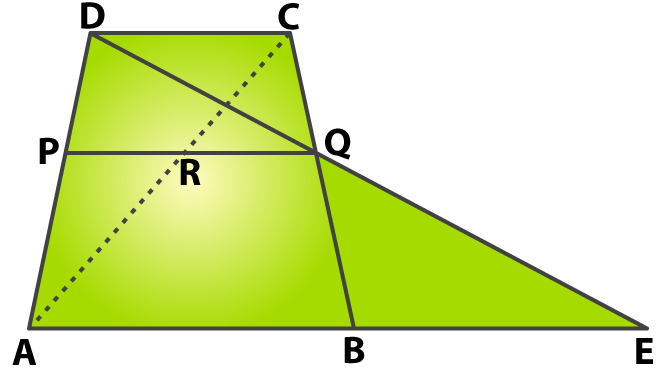Solution:

(i) In △ QCD and △ QBE

We know that ∠ DQC and ∠ BQE are vertically opposite angles

So we get

∠ DQC = ∠ BQE

From the figure we know that Q is the midpoint of BC

It can be written as

CQ = BQ

We know that AE || DC and BC is a transversal

From the figure we know that ∠ QDC and ∠ QEB are alternate angles

∠ QDC = ∠ QEB

By ASA congruence criterion

△ QCD ≅ △ QBE

DQ = QE (c. p. c. t)

Therefore, it is proved that DQ = QE

(ii) Based on the midpoint theorem

We know that PQ || AE

From the figure we know that AB is a part of AE

So we have PQ || AB

We know that the intercepts on AD are made by the lines AB, PQ and DC

So we get PQ || AB || DC

We know that PR is a part of PQ and is parallel to AB

So we get PR || AB || DC

Therefore, it is proved that PR || AB.

(iii) From the figure we know that the lines PR, AB and DC are cut by AC and AD respectively.

Based on the intercept theorem we know that AR = RC.

Therefore, it is proved that AR = RC.

8. In the adjoining figure, AD is a median of △ ABC and DE || BA. Show that BE is also a median of △ ABC.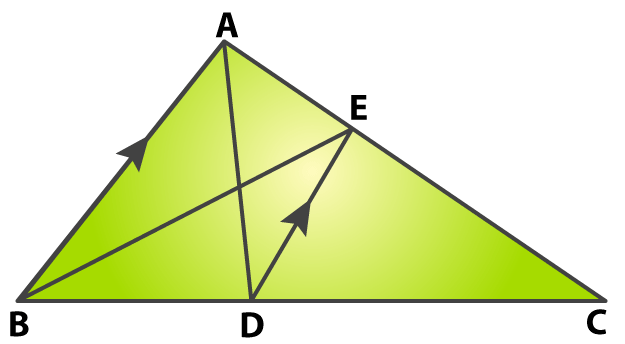Solution:

Consider △ ABC

It is given that DE || AB

We know that D is the midpoint of BC

Based on the midpoint theorem we know that

E is the midpoint of AC

So we know that BE is the median of △ ABC drawn through B.

Therefore, it is proved that BE is also a median of △ ABC.

9. In the adjoining figure, AD and BE are the medians of △ ABC and DF || BE. Show that CF = ¼ AC.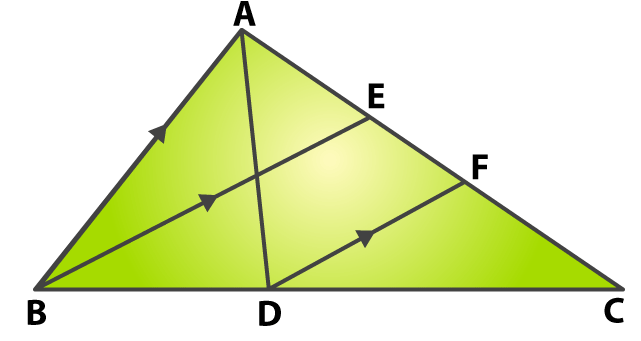Solution:

Consider △ CBE

From the figure we know that D is the midpoint of BC and DF is parallel to BE.

By using the midpoint theorem

We know that F is the midpoint of EC.

It can be written as

CF = ½ EC

We know that BE is the median through B

So we get

CF = ½ [(1/2) AC]

CF = ¼ AC

Therefore, it is proved that CF = ¼ AC.

10. Prove that the line segments joining the middle points of the sides of a triangle divide it into four congruent triangles.

Solution: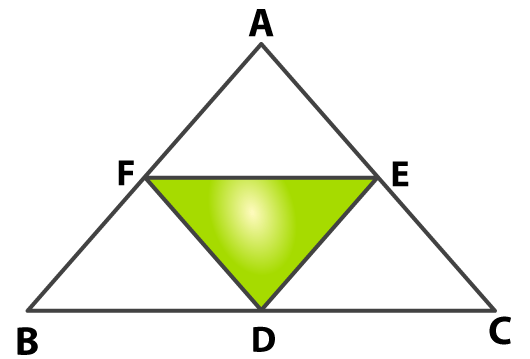From the figure we know that F and E are the mid points of AB and AC

Based on the mid-point theorem

EF = ½ BC

In the same way

FD = ½ AC and ED = ½ AB

Consider △ AFE and △ BFD

We know that AF = FB

Based on the midpoint theorem

FE = ½ BC = BD

FD = ½ AC = AE

By SSS congruence criterion

△ AFE ≅ △ BFD

Consider △ BFD and △ FED

We know that FE || BC

So we get FE || BD and AB || ED

Using the midpoint theorem

FB || ED

Hence, BDEF is a parallelogram

So we know that FD is a diagonal which divides the parallelogram into two congruent triangles

△ BFD ≅ △ FED

In the same way we can prove that FECD is a parallelogram

△ FED ≅ △ EDC

So we know that △ BFD, △ FDE, △ FED and △ EDC are congruent to each other.

Therefore, it is proved that the line segments joining the middle points of the sides of a triangle divide it into four congruent triangles.

11. In the adjoining figure, D, E, F are the midpoints of the sides BC, CA and AB respectively, of △ ABC. Show that ∠ EDF = ∠ A, ∠ DEF = ∠ B and ∠ DFE = ∠ C.Solution:

Consider △ AFE and △ DFE

Using the midpoint theorem

AF = ½ AB = ED

AE = ½ AC = FD

FE is common i.e. FE = EF

By SSS congruence criterion

△ AFE ≅ △ DFE

∠ A = ∠ FDE (c. p. c. t)

In the same way we get ∠ B = ∠ DEF and ∠ C= ∠ DFE.

Therefore, it is proved that ∠ EDF = ∠ A, ∠ DEF = ∠ B and ∠ DFE = ∠ C.

12. Show that the quadrilateral formed by joining the midpoints of the pairs of adjacent sides of a rectangle is a rhombus.

Solution: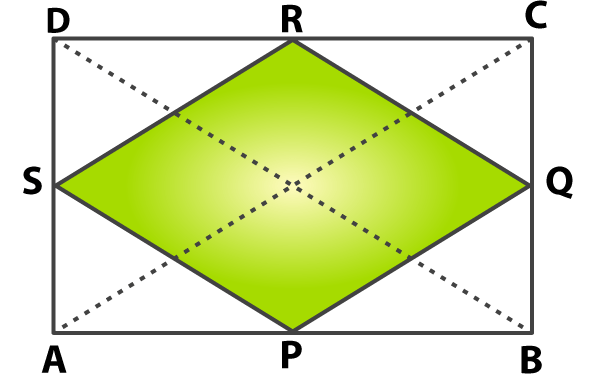Consider △ ABC

We know that P and Q are the midpoints of AB and BC

Based on the midpoint theorem

We know that PQ || AC and PQ = ½ AC

Based on the midpoint theorem

We know that RS || AC and RS = ½ AC

It can be written as PQ || RS and

PQ = RS = ½ AC ……. (1)

We know that P and S are the midpoints of AB and AD

Based on the midpoint theorem

We know that PS || BD and PS = ½ DB

Consider △ BCD

We know that RQ || BD and RQ = ½ DB

It can be written as PS || RQ and

PS = RQ = ½ DB ……… (2)

We know that the diagonals of a rectangle are equal

It can be written as

AC = BD ………. (3)

Comparing equations (1), (2) and (3)

We know that

PQ || RS and PS || RQ

So we get

PQ = QR = RS = SP

Therefore, it is proved that the quadrilateral formed by joining the midpoints of the pairs of adjacent sides of a rectangle is a rhombus.

13. Show that the quadrilateral formed by joining the midpoints of the pairs of adjacent sides of a rhombus is a rectangle.

Solution: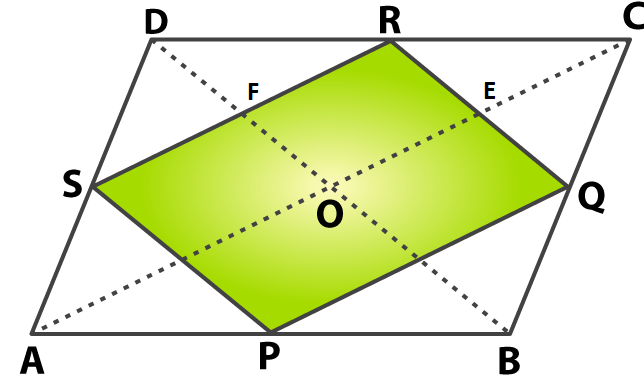Consider △ ABC

We know that P and Q are the mid points of AB and BC

By using the midpoint theorem

We know that PQ || AC and PQ = ½ AC

We know that RS || AC and RS = ½ AC

It can be written as PQ || RS and

PR = RS = ½ AC ……. (1)

We know that P and S are the mid points of AB and AD

Based on the midpoint theorem

We know that PS || BD and PS = ½ DB

Consider △ BCD

We know that RQ || BD and RQ = ½ DB

It can be written as PS || RQ and

PS = RQ = ½ DB ……. (2)

By considering equations (1) and (2)

The diagonals intersects at right angles in a rhombus

So we get

∠ EQF = 90o

We know that RQ || DB

So we get RE || FO

In the same way SR || AC

So we get FR || OE

So we know that OERF is a parallelogram.

We know that the opposite angles are equal in a parallelogram

So we get

∠ FRE = ∠ EOF = 90o

So we know that PQRS is a parallelogram having ∠ R = 90o

Therefore, it is proved that the quadrilateral formed by joining the midpoints of the pairs of adjacent sides of a rhombus is a rectangle.

14. Show that the quadrilateral formed by joining the midpoints of the pairs of adjacent sides of a square is a square.

Solution:

Consider △ ABC

We know that E and F are the midpoints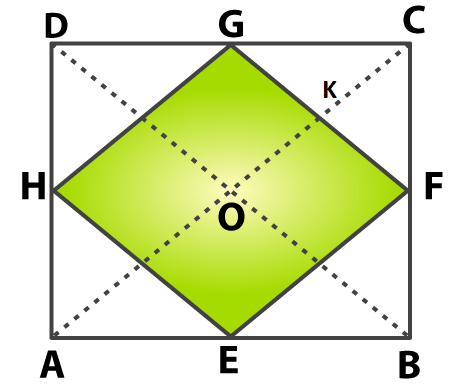Based on the midpoint theorem

We know that EF || AC and EF = ½ AC

We know that H and G are the midpoints

Based on the midpoint theorem

We know that HG || AC and HG = ½ AC

So we get EF || HG and

EF = HG = ½ AC ……….. (1)

We know that H and E are the midpoints

Based on the midpoint theorem

We know that HE || BD and HE = ½ BD

Consider △ BCD

We know that G and F are the midpoints

Based on the midpoint theorem

We know that GF || BD and GF = ½ BD

So we get HE || GF and

HE = GF = ½ BD …….. (2)

We know that the diagonals of a square are equal

So we get

AC = BD …….. (3)

By using equations (1), (2) and (3)

So we get GF || BD and HE || GF

We have

EF = GH = GH = HE

We know that EFGH is a rhombus

From the figure we know that the diagonals of a square are equal and intersect at right angles

∠ DOC = 90o

We know that the sum of adjacent angles of parallelogram is 180o

It can be written as

∠ DOC + ∠ GKO = 180o

By substituting the values

90o + ∠ GKO = 180o

On further calculation

∠ GKO = 180o – 90o

By subtraction

∠ GKO = 90o

From the figure we know that ∠ GKO and ∠ EFG are corresponding angles

We get ∠ GKO = ∠ EFG = 90o

We know that ∠ EFG = 90o

Hence, EFGH is a square.

Therefore, it is proved that the quadrilateral formed by joining the midpoints of the pairs of adjacent sides of a square is a square.

15. Prove that the line segments joining the midpoints of opposite sides of a quadrilateral bisect each other.

Solution:

Construct lines KH, BD and GL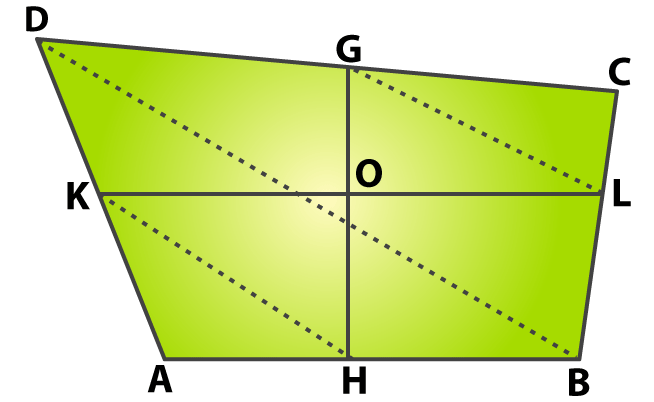We know that K and H are the midpoints of AD and AB

Consider △ ABD

Using the mid-point theorem

We get

KH = ½ BD

Consider △ CBD

Based on the midpoint theorem

GL = ½ BD

So we get

KH = GL

Consider △ KOH and △ GOL

We know that KH = GL

From the figure we know that alternate angles are equal

So we get

∠ OKH = ∠ GLO and ∠ OHK = ∠ OGL

By SAS congruence criterion

△ KOH ≅ △ GOL

OG = OH and OK = OL (c. p. c. t)

So we know that GH and KL bisect each other.

Therefore, it is proved that the line segments joining the midpoints of opposite sides of a quadrilateral bisect each other.

16. The diagonals of a quadrilateral ABCD are equal. Prove that the quadrilateral formed by joining the midpoints of its sides is a rhombus.

Solution:Consider △ ABC

We know that P and Q are the midpoints of AB and BC

So we get PQ || AC and

PQ = ½ AC …… (1)

Consider △ BCD

We know that Q and R are the midpoints of BC and CD

So we get QR || BD and

QR = ½ BD ……. (2)

We know that S and R are the midpoints of AD and CD

So we get RS || AC and

RS = ½ AC ……. (3)

Consider △ ABD

We know that P and S are the midpoints of AB and AD

So we get SP || BD and

SP = ½ BD ……. (4)

Using all the equations

PQ || RS and QR || SP

Thus, PQRS is a parallelogram

It is given AC = BD

We can write it as

½ AC = ½ BD

From the equations we get

PQ = QR = RS = SP

PQRS is a rhombus

Therefore, it is proved that the quadrilateral formed by joining the midpoints of its sides is a rhombus.

17. The diagonals of a quadrilateral ABCD are perpendicular to each other. Prove that the quadrilateral formed by joining the midpoints of its sides is a rectangle.

Solution: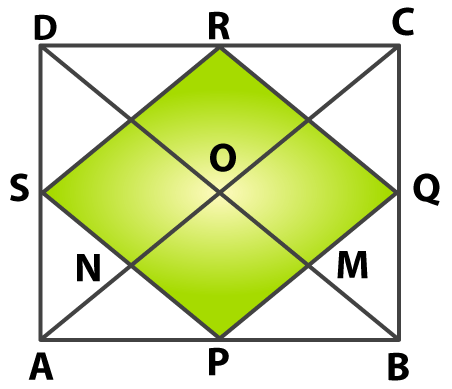Consider △ ABC

We know that P and Q are the midpoints of AB and BC

So we get PQ || AC and

PQ = ½ AC …… (1)

We know that R and S are the midpoints of CD and AD

So we get RS || AC and

RS = ½ AC …….. (2)

Using the equations

We get PQ || RS and PQ = RS

We know that a pair of opposite sides are equal in a parallelogram

We know that AC and BD are the diagonals intersecting at point O

Consider △ ABD

We know that P and S are the midpoints of AD and AB

So we get

PS || BD

It can be written as

PN || MO

Based on equation (1)

We get

PM || NO

So we know that PM || NO and PN || MO

We know that opposite angles are equal in a parallelogram

∠ MPN = ∠ MON

We know that

∠ BOA = ∠ MON

So we get

∠ MPN = ∠ BOA

We know that AC ⊥ BD and ∠ BOA = 90o

It can be written as

∠ MPN = 90o

So we get

∠ QPS = 90o

So we know that PQRS is a parallelogram with ∠ QPS = 90o

Therefore, it is proved that the quadrilateral formed by joining the midpoints of its sides is a rectangle.

18. The midpoints of the sides AB, BC, CD and DA of a quadrilateral ABCD are joined to form a quadrilateral. If AC = BD and AC ⊥ BD then prove that the quadrilateral formed is a square.

Solution:Consider △ ABC

We know that P and Q are the midpoints of AB and BC

So we get PQ || AC and

PQ = ½ AC …… (1)

Consider △ BCD

We know that Q and R are the midpoints of BC and CD

So we get QR || BD and

QR = ½ BD ……. (2)

Consider △ ACD

We know that S and R are the midpoints of AD and CD

So we get RS || AC and

RS = ½ AC …….. (3)

Consider △ ABD

We know that P and S are the midpoints of AB and AD

So we get SP || BD and

SP = ½ BD ……. (4)

Consider all the equations

PQ || RS and QR || SP

Hence, PQRS is a parallelogram

It is given that AC = BD

It can be written as

½ AC = ½ BD

So we get

PQ = QR = RS = SP

We know that AC and BD intersect at point O

So we get PS || BD

PN || MO

Based on equation (1)

We get PQ || AC

PM || NO

We know that the opposite angles are equal in a parallelogram

∠ MPN = ∠ MON

We know that ∠ BOA = ∠ MON

So we get

∠ MPN = ∠ BOA

We know that AC ⊥ BD and ∠ BOA = 90o

So we get

∠ MPN = 90o

It can be written as

∠ QPS = 90o

We know that PQ = QR = RS = SP

Therefore, it is proved that PQRS is a square.

### Access other exercise solutions of Class 9 Maths Chapter 10: Quadrilaterals

Exercise 10A Solutions 10 Questions

Exercise 10B Solutions 27 Questions

### RS Aggarwal Solutions Class 9 Maths Chapter 10 – Quadrilaterals Exercise 10C

RS Aggarwal Solutions Class 9 Maths Chapter 10 Quadrilaterals Exercise 10C has two Midpoint theorems and exercise problems which are solved using them.

### Key features of RS Aggarwal Solutions for Class 9 Maths Chapter 10: Quadrilaterals Exercise 10C

• The main objective is to make education activity based rather than mugging up of answers.
• The solutions are solved using the latest CBSE exam pattern which gives an idea about which topic carries maximum marks.
• Difficult problems are provided with pictures which makes it easy for the students to study effectively.
• Important formulas and theorems are explained in a structured manner as it has more weightage in the board exam.